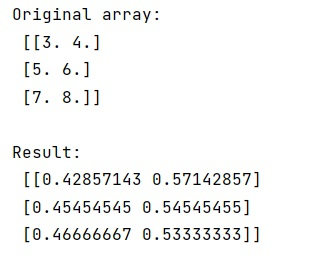# NumPy: Divide row by row sum

Learn, how to divide row by sum of the numpy array?
Submitted by Pranit Sharma, on January 24, 2023

NumPy is an abbreviated form of Numerical Python. It is used for different types of scientific operations in python. Numpy is a vast library in python which is used for almost every kind of scientific or mathematical operation. It is itself an array which is a collection of various methods and functions for processing the arrays.

## Dividing row by row sum

Suppose that we are given a NumPy array and we need to divide this NumPy array's row by the sum of all the values in that row.

The easiest approach to solve this problem is to divide the array by the sum of the specified row by defining the axis as 1 and slice it by using None.

Let us understand with the help of an example,

## Python code to divide row by row sum

```# Import numpy
import numpy as np

# Import math
import math

# Creating a numpy array
arr = np.array([[3., 4.],[5., 6.],[7., 8.]])

# Display original array
print("Original array:\n",arr,"\n")

# dividing the row by its sum
res = arr/arr.sum(axis=1)[:,None]

# Display result
print("Result:\n",res,"\n")
```

Output:What's New (MCQs)

Top Interview Coding Problems/Challenges!

IncludeHelp's Blogs

Languages: » C » C++ » C++ STL » Java » Data Structure » C#.Net » Android » Kotlin » SQL
Web Technologies: » PHP » Python » JavaScript » CSS » Ajax » Node.js » Web programming/HTML
Solved programs: » C » C++ » DS » Java » C#
Aptitude que. & ans.: » C » C++ » Java » DBMS
Interview que. & ans.: » C » Embedded C » Java » SEO » HR
CS Subjects: » CS Basics » O.S. » Networks » DBMS » Embedded Systems » Cloud Computing
» Machine learning » CS Organizations » Linux » DOS
More: » Articles » Puzzles » News/Updates